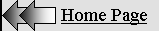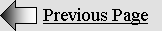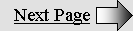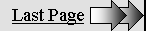LORENTZIAN  RELATIVITY

Page 2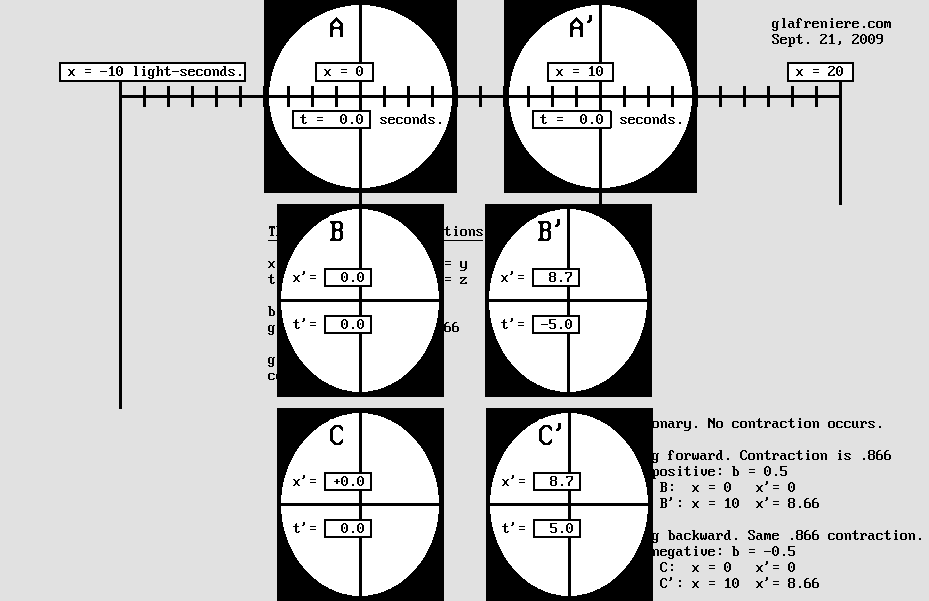Relativity is surprisingly simple.

It just requires elementary mathematical operations.

However, it also requires reasonable knowledge of the Lorentz Transformations.

This is why the above diagram should help.

 So many experiments. The goal of the previous page was to expose the real effects of the Lorentz transformations, that is to say: a length contraction, a local time, and a slower behavior. Because of these, a moving observer cannot find out whether or not he is moving with respect to the aether. This is called Lorentzian Relativity. One may now carefully examine these many experiments where Lorentz's Relativity holds true. Fortunately, they do not require complicated formulas. So many flawless demonstrations put together finally builds solid proof that Lorentz was right. 1. The absence of transverse contraction prevents the observer from detecting his absolute motion. 2. The radar echoes simulate or dissimulate the axial contraction. 3. The clock synchronization procedure ends up with a time shift. 4. The absolute motion causes the slowing down of all mechanisms. 5. The contraction cannot be detected by any means such as optical imaging or triangulation.  6. The speed of light seems to be the same in all frames of reference. 7. There are no means to detect that clocks are running slower. 8. The Michelson interferometer cannot detect its absolute motion because it undergoes a contraction. 9. The beam splitter angle must match the interferometer contraction in order to reflect correctly the light beam. 10. Bradley's stellar aberration cannot reveal the Earth's absolute motion. 11. The Fresnel-Fraunhofer diffraction pattern cannot reveal the absolute motion. 12. Light undergoes a symmetrical Doppler effect, so that relative motion only can be detected. 13. Any speed beyond the speed of light is impossible. 14. The relative speed between two material bodies may approach twice the speed of lignt. 15. Standing waves contract according to Lorentz on the condition that the frequency also slows down according to Lorentz. 16. Lorentzian Relativity remains consistent in the presence of an unlimited number of material bodies. 17. There is no General Relativity because gravity is not linked to Relativity. 18. The Lorentz transformations lead to Relativity but they also extend well beyond it.     The list is not exhaustive. For example, it is a well known fact that the Doppler effect is not perceivable if both the emitter and the observer are moving along in the same frame of reference, which is the case most of the time. Such elementary propositions do not need further analysis. One may also imagine very complex ones, yet so elaborate that adding these to the list would only add confusion. It's better to keep things simple. Below is a video which reproduces more clearly the scene shown above. It should be helpful in understanding why observer B in particular is fooled by the Lorentz transformations. Lorentz_Transformations.mkv Pay attention to the radio signal emitted by B. It is represented by the red circle growing at the speed of light. Naturally, its center remains stationary with respect to the aether. Upon reception of the signal, observer B' emits a new signal (the green circle) towards B who finally receives it. On the one hand, the recorded delay apparently indicates that the distance to B' is 10 light-seconds. On the other hand, the same signal also indicates that the B' clock is perfectly synchronized because it includes the time when B' emitted it. However, the correct distance is 8.66 light-seconds instead and additionally, a 5 second time shift occurred as a result of this clock synchronization procedure. B is wrong because of the Lorentz transformations. Attempts to verify his absolute speed always fail. Absolute motion is unverifiable.

1. THE ABSENCE OF TRANSVERSE CONTRACTION PREVENTS THE OBSERVER TO FINDING OUT HIS ABSOLUTE SPEED.

 The electron is made of standing waves. If it moves, its waves contract on the translation axis, but they don't on transverse y and z axes. This is consistent with the Lorentz transformations: y'  =  y z'  =  z Because the electron frequency slows down according to Lorentz's g factor, its transverse wavelength remains unchanged at any speed in such a way that matter, which is made of electrons, never contracts transversally. Transverse distances do not contract either because all transverse forces responsible for matter mechanics remain stable too. It is also the case for light and radio waves because they are emitted by electrons. Consequently, the observer can no longer find out his velocity with respect to the aether by observing changes in transverse distances, transverse light wavelength or transverse forces. This is a very rare case where direct and flawless comparison is possible between two systems whose speed differ. So let us examine this interesting situation.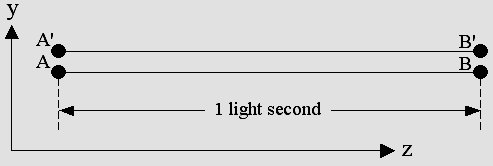Please note that both y and z are transverse axes. Observers A and B are stationary with respect to the aether. Motion for A' and B' is parallel to the orthogonal x axis (not visible on this plane). The distance is the same for both systems: one light-second or 300 000 km (186,000 miles). A, A' and B, B' may come very close together and act like a measuring rod.  There is no time shift on this plane, so they can simultaneously check that no contraction occurs.     Because wavefronts are tilted according to the theta angle on the moving transverse plane including A' and B' on the above diagram, light or radio waves seem to propagate more slowly according to g. However, the time needed for a radio signal to travel a transverse distance follows exactly the slower hours according to g: The waves' traveling time along a transverse axis is:  1 / g slower. Surprisingly, if A' and B' want to check the distance between each other using a radar or a radio signal, they obtain the correct value in spite of two serious errors. Their clocks display slower seconds but the radio signal velocity is also slower according to the same g factor. Thus they can still verify that the distance to the opposite moving observer is one light-second. They can also measure y or z distances correctly using radio wavelength absolute units because transverse wavelength never changes. So the very first condition for Relativity to be true is the absence of transverse contraction. Otherwise, A and B would clearly observe that A' and B' are nearer while A' and B' would instead observe that A and B are more distant. There would be no reciprocity any more and their absolute speed with respect to the aether would become measurable. However, Lorentz and Poincaré were not aware that matter is made of waves. They had to laboriously use Maxwell's equations. So they wrote page upon page of equations on magnetic fields, electric fields, permittivity, permeability, light propagating through dielectrics. They had to explain the Fizeau experiment, the Michelson experiment, Bradley's stellar aberration, etc. The point is that they were not fully aware that they were just dealing with the Doppler effect. This is quite surprising because they were studying how electromagnetic waves should behave in the presence of motion. What's more, the standing wave contraction phenomenon was totally unknown in 1900. It was discovered by Mr. Ivanov around 1990 only. But they succeeded anyway. Poincaré wrote that, using the "least action principle", he finally found that Voigt's constant should be equal to 1. But he very clearly adds in two different books that Lorentz had already found this equality using the "trial and error method" (par tâtonnements). The Lorentz transformations are just a special case of Voigt's equations where the constant k is 1 and where the slower frequency leads to the absence of transverse contraction. So this useless and bulky constant could be removed and Lorentz's equation set became simpler. Instead of Voigt's y' = k y; z' = k z, Lorentz found that y' = y; z' = z, which means that matter does not contract transversally.

2. THE RADAR ECHOES SIMULATE OR DISSIMULATE THE AXIAL CONTRACTION.

 Things are getting worse when observers B and B' below try to measure the distance between each other using light or radio signals.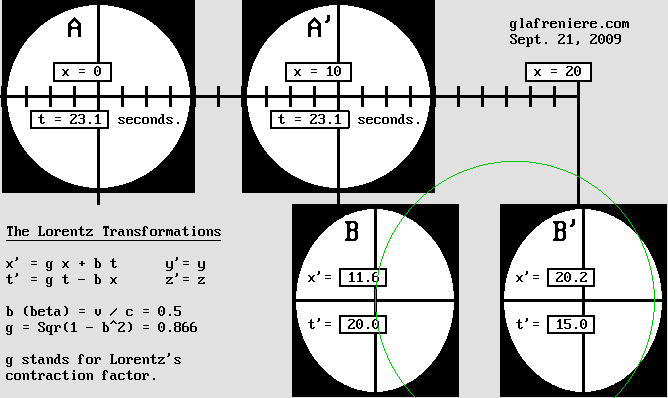Observer B receives the radar echo (the green circle) after 20 slow seconds according to his clock. This 20 second delay erroneously indicates that the distance to B' is 10 light-seconds. The radar echo dissimulates the contracted distance, which is only: 20.21 – 11.55 = 8.66 light-seconds. The Lorentz transformations for observer B : x' = g x + b t = (0.866 * 0) + (0.5 * 23.1) =  11.55 light-seconds. t' = g t – b x = (0.866 * 23.1) – (0.5 * 0) = 20 slow seconds. The Lorentz transformations for observer B' : x' = g x + b t = (0.866 * 10) + (0.5 * 17.33) =  20.21 light-seconds. t' = g t – b x = (0.866 * 23.1) – (0.5 * 10) = 15 slow seconds.      The problem is that they are fooled for three different reasons. Firstly, they think that they are stationary. Secondly, they are unaware that their clocks display slower seconds. And thirdly, the mean speed of a radar signal on a round trip is slower according to g squared (see No. 4 below). Let's suppose that observer B wants to make sure that the distance to B' is 10 light-seconds. The radar is the most accurate instrument for this. The radar signal is emitted exactly when he meets A so that their clocks are synchronized to 0 second. In a stationary system, the delay for a 20 light-second total distance should be 20 seconds. However, because B and B' are moving, the distance is actually: 2 * 8.66 = 17.32 light-seconds. The mean speed of the radar signal is also slower according to g squared = 0.75 c. The time for the radar signal to perform the round trip is: 17.32 / 0.75 = 23.09 absolute seconds. And finally, the B clock which runs more slowly records 0.866 * 23.09 = 20 slow seconds. Amazingly, the delay is 20 seconds in both frames of reference. Such a result apparently confirms Einstein's postulate:    "The speed of light is the same in all inertial frames of reference." Let's put it clearly: the speed of light is not the same in all inertial frames of reference. It indeed appears to be constant, but it is an illusion leading to a mystification. B is now quite sure that the distance to B' is 10 light-seconds, but he is definitely wrong because the slower radar echo together with the slower seconds hides the contraction. Simulating the contraction. Inversely, the radar echo may simulate the contraction as well. If observer B measures the A to A' distance, he faces a similar trap. A and A' are stationary with respect to the aether and hence, the speed of a radar signal between them is truly the speed of light. That is why the delay between the two echoes is obviously 20 seconds for a 10 light-second distance. All observers must be positioned on the displacement axis. Now, suppose that B is moving away from A and A'. Then the virtual Doppler effect increases the delay according to: 1 + beta = 1.5 so that it is instead: 20 * 1.5 = 30 seconds. Moreover, the B clock will record : 30 * 0.866 = 25.98 slower seconds. This is what really happens, but B considers that A and A' are moving away from him. He must interpret this result his own way. Because they are moving away, he expects that the radar delay will be longer according to the Doppler effect, that is to say: 1 + beta = 1.5. He is entitled to presume that the delay is: 25.98 / 1.5  = 17.32 seconds instead. This result indicates that the A to A' distance is only: 17.32 / 2 = 8.66 light-seconds. If B was moving in the opposite direction, the Doppler effect would be reversed according to: 1 – beta = 0.5 and his conclusion would be the same: the A to A' distance is contracted. In addition, B observes that his clock indicates 17.32 seconds when he meets A'. Because he thinks that A and A' are moving at half of the speed of light, this additional verification confirms that the A to A' distance is 8.66 light-seconds. In spite of two carefully conducted verifications, B is wrong. Once again, he is fooled because this time, the radar simulates the Lorentz contraction.  Amazingly, B observes that the A-A' system is contracted and that his own B-B' system is not. A and B are unable to reach consensus by comparing their situation because their observations are perfectly symmetric. It becomes impossible to determine who is really moving with respect to the aether. Those phenomena seem relative, but actually they are absolute. Clearly, A observes the situation correctly but B is totally wrong. There is no symmetry. Train and tunnel paradox. The train and tunnel paradox was one of Einstein's unsolvable problems. Thanks to Lorentzian Relativity, which postulates that the ether exists and that the Lorentz transformations really transform matter, one may easily solve the paradox by elementary calculation. The answer is: The train or the tunnel may be longer, but who knows which one? The point is that both situations are not possible simultaneously. Only one observer is recording the situation correctly while the other one is fooled. Knowing that, there is no paradox any more. Incertitude remains, though.

3. THE CLOCK SYNCHRONIZATION PROCEDURE ENDS UP WITH A TIME SHIFT

 As seen above, observer B could synchronize his clock according to A when he met him on the same coordinate: x = x' = 0; t = t' = 0. The distance between each other being negligible, the Doppler effect is no longer fooling him. At this very moment, observer B transmits a signal to B' in order to inform him that the time was reset to zero second. Because he erroneously measured that the distance to B is 10 light-seconds, B' adjusts his clock to 10 seconds upon reception of the signal (he thinks that the B clock should now display 10 seconds because of the delay). However, a problem arises because the signal speed is reduced to: 1 – beta = 0.5 c if the signal is emitted forward. It is a mere consequence of the Doppler effect or more exactly, of his displacement. Thus, the actual time delay is: t = 8.66 / (1 – 0.5) = 17.33 seconds but B' is totally unaware of this anomaly. Again, note that for observer B, x = 0 while for B' it is x = 10 instead. We also found that t = 17.33 when B' receives the signal from B. Knowing this, the Lorentz reversed equations allow us to obtain the new x' position for B and B'. We will also find what time their clocks are displaying: Observer B:  x' = g x + b t = (0.866 * 0) + (0.5 * 17.33) =  8.66 light-seconds. t' = g t – b x = (0.866 * 17.33) – (0.5 * 0) = 15 slow seconds. Observer B': x' = g x + b t = (0.866 * 10) + (0.5 * 17.33) =  17.33 light-seconds. t' = g t – b x = (0.866 * 17.33) – (0.5 * 10) = 10 slow seconds.     These results are displayed in the image below. The B clock is ticking slower seconds and hence the correct time t = 17.33 was reduced to 15 seconds. The interesting point is that B' is receiving the signal from B (represented by the red curve below) at this very moment. B' adjusts his own clock to 10 seconds as explained above. That is why his clock will permanently display a –5 second time shift (according to : – beta * x = – 0.5 * 10) with respect to B. Please note that B' does what appears to be the correct thing to do. The equation simply predicts his incorrect behavior and the time t' is obviously incorrect. This is consistent with Lorentz's opinion in 1904: "My local time t' must be regarded as no more than an auxiliary mathematical quantity". And because this calculus is based on the Doppler effect and on the existence of the aether, it proves to be a spectacular argument in favor of Lorentz's Relativity.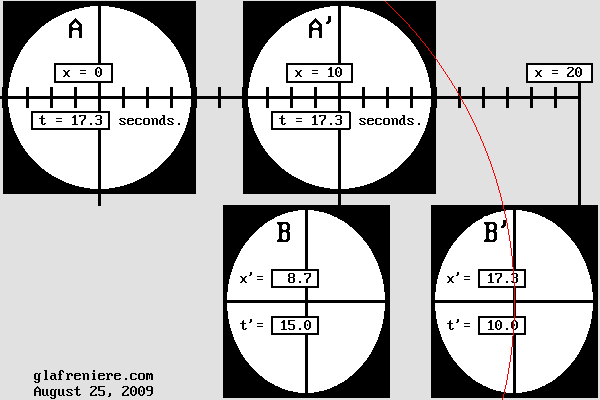When B' receives the synchronization signal (the red curve), the absolute time according to A and A' is 17.33 seconds. The B clock shows 15 slow seconds instead: 17.33 * 0.866 = 15. However, B' adjusts his clock to 10 seconds so that a permanent –5 second time shift takes place with respect to B. Please note that unlike the t' slower time, all x and x' coordinates are absolute. They are referring to a Cartesian frame of reference which is stationary with respect to the aether.     When B' receives the signal, he immediately emits another one towards B in order to inform him that his clock was adjusted to 10 seconds. This signal is represented by the green curve shown below, which is finally received by B after 23.1 absolute seconds or 20 slow seconds. It is well known that the signal duration on a round trip (17.32 light-seconds here) is increased according to 1 / g^2: 17.32 / 0.866^2 = 23.1. Then, thanks to my reversed formulas, it becomes possible to verify that the B clock must show 20 seconds while the B' one shows 15 seconds instead, which is consistent with the –5 second time shift.Observer B : x' = g x + b t = (0.866 * 0) + (0.5 * 23.1) =  11.55 light-seconds. t' = g t – b x = (0.866 * 23.1) – (0.5 * 0) = 20 slow seconds. Observer B': x' = g x + b t = (0.866 * 10) + (0.5 * 17.33) =  20.21 light-seconds. t' = g t – b x = (0.866 * 23.1) – (0.5 * 10) = 15 slow seconds.     On the one hand, observer B has timed that the total duration was 20 seconds, which apparently confirms that the distance to B' is 10 light-seconds. However, the correct distance is 8.66 light-seconds. On the other hand, the signal from B' appears to confirm that the B' clock is perfectly synchronized with his own clock because its duration was apparently 10 seconds. But it is not. According to B and B', all happens as though they were perfectly stationary with respect to the aether. There is no evidence that a Doppler effect occurred because of three superimposed effects: the contraction, the slower clocks and the time shift. Finally, because A and A' are aware that they might have been fooled in the same manner, none of all those observers can say for sure which one is truly stationary. Lorentz's Relativity is absolutely fantastic!

4. MECHANISMS IN MOTION RUN MORE SLOWLY.

 Speaking about time dilation is ridiculous. The clock mechanism runs more slowly instead. All mechanisms run more slowly according to their velocity with respect to the aether because their individual parts must travel an increased absolute distance. The cause of such a displacement is the radiation pressure exerted by waves incoming from fields of force, which are made of standing waves undergoing the Doppler effect. The speed of all those waves, including light and radio waves, is constant with respect to the aether. The goal is to figure out by how much their absolute individual displacement is increased, in order to estimate the time needed for the waves to travel the resulting longer distance. This was Michelson's great idea. He actually discovered that the traveling time should be longer along the displacement axis x then on transverse axes y and z. This idea allowed him to build his famous interferometer, considering that the difference should become visible thanks to interferences. The wave behavior may easily be compared to that of planes flying in the presence of a strong wind. Below, a plane is flying between A and B on a round trip. It will represent the transverse axis y. The other plane is performing a similar round trip along the AC or x axis, which will be that of the wind. Both distances are equal and the plane speed is postulated to be constant in order to simulate the constant speed of light. The first test is executed in the absence of wind.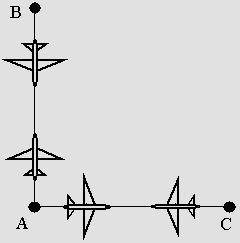In the absence of wind, the distance being the same, the flying time is also the same.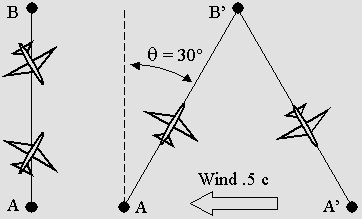Here, the wind speed is 50% of the plane speed: beta = 0.5. On the left hand side, the planes are observed from the ground and they appear tilted according to the theta angle below. On the right hand side, they are represented in their absolute frame of reference, which is that of the air. Clearly, the absolute distance and the round trip duration are increased according to the theta angle: theta = ArcSin(beta) = 30 ° g = Sqr(1 – beta^2) = Cos(theta) = 0.866 The round trip duration is increased according to:  1 / g = 1.1547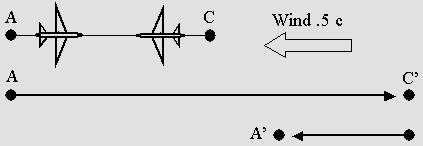Now, the wind axis coincides with that of motion. In this case, the round trip duration is increased according to 1 / g 2 = 1.3333     In order to obtain the same duration on a round trip, it becomes clear that the A to C distance must be reduced according to g. Then both planes perform the test during the same period of time, which is 1.1547 longer according to g. Because clocks tick more slowly according to the same g factor, the recorded time remains invariable in any frame of reference. This hypothesis was called the "FitzGerald-Lorentz contraction" and it is the basis of Lorentzian Relativity: x' = g x without the translation. The Michelson interferometer contracts in such a way that interferences no longer occur. Michelson was unaware of this. Because matter contracts this way, his apparatus becomes unable to measure the aether wind. This is not a joke. Today, most scientists consider that Lorentz's hypothesis was too strange and unbelievable to be true. However, I am working on a new science which I call "Motion Optics". It is based on the amazing Delmotte-Marcotte virtual medium. Up to now, the results have been dramatically consistent with the Lorentz transformations. The video below should force physicists and especially opticians to drastically review their judgment:  Michelson_Interferometer.5c.mkv The plane behavior simulates pretty well that of any other mechanism. Each piece of the ensemble must travel a longer distance which requires more time even though the contraction applies. For example, a fast moving clock pendulum should oscillate more slowly so that this clock would display slower, hence inaccurate hours with respect to an unmoving one: t' = g t without the time shift. Standing waves also behave this way on the condition that the frequency is reduced in order to allow the wave fronts to perform the round trip between nodes and antinodes in any direction. Please bear in mind that standing waves contract (see No. 15 below). Surely, matter contracts because it is made up of standing waves. Adding the translation and the time shift, one finally obtains my reversed formulas: x' = g x + b t t'  =  g t – b x

5. THE CONTRACTION CANNOT BE REVEALED BY ANY MEANS INCLUDING OPTICAL IMAGING OR TRIANGULATION.

 Alice in Wonderland. The relative point of view becomes obvious when Alice sees the house becoming smaller. She may actually become taller as well. But what if both Alice and the house become taller? Then she would become unable to observe any difference on the condition that, ultimately, the whole universe also becomes taller. In this case, distances on the three Cartesian axes are modified. Now, the goal is to show that the observer still cannot observe any difference if distances on one axis only are modified, which is Lorentz's postulate. It was shown above that the axial contraction could not be measured using radar signals. Here, we will focus on optical and geometric verifications only.  Descartes' formula. Considering that the observer's eyes contract along the motion axis only, basic optical laws including Descartes' formula below are still valid. The eye and its spherical retina may be compared to the pinhole camera, whose image becomes distortion-free on a spherical surface (one obtains an azimutal projection - not Mercator - if it is cylindrical). If contraction occurs, this device can still produce an image whose proportions are preserved. Descartes showed that the entrance pupil, which is the pinhole here, was an important element of a camera. It is the intermediate point whose distance to the internal image  (L1) and to the object (L2) in an imaging device which firstly allows one to know the magnification ratio X of the system. It is given by: X = L1 / L2 Below, on the left hand side, distances are: L1 = 1 and L2 = 4 in both cases in spite of the transverse contraction for the upper situation. This indicates that the image will be reduced to 0.25 times the original object, which is an identical pinhole camera (the image will be 4 times smaller). On the right hand side, because of longitudinal contraction, distances are: L1 = 0.5 et L2 = 2. Thus, the magnification ratio is always 0.25 in all three situations.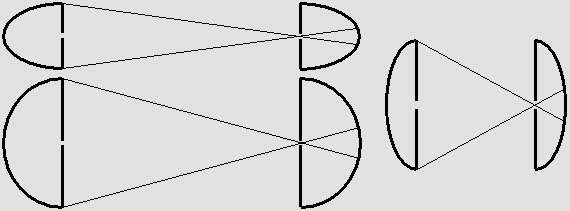Whatever the contraction, the pinhole camera produces an image whose proportions are conserved.     The new Motion Optics. Motion optics is a brand new science which I am elaborating in order to show that the Lorentz transformations must occur in order to preserve the accuracy of any moving optical system. This includes a satellite parabola which is emitting waves towards the earth, given the fact that they are obviously suffering from the Doppler effect. The video below shows that the moving observer cannot notice any anomaly, which is the goal here. It reproduces the whole Bradley aberration process. I even added a transformation using my Time Scanner, which can show how the scene would appear as seen by the contracted observer moving along with the main contracted parabola. Please note that the two small parabolas do not move at the same speed so that the contraction differs. One is moving slower than the observer and the other one is moving faster. However, the two focal points still appear identical to the observer's eyes. But what's more, it is a flawless demonstration that Lorentz's predictions about matter contraction must occur. It is a compulsory condition for optical phenomena to remain consistent in a moving device. If the contraction does not occur, the focus point suffers from severe distortion. Bradley_Aberration.5c_Scan.mkv The geometer calculus. The moving geometer may use a contracted protractor template in order to measure angles. Because angles in any moving material structure are contracted in the same manner, any measuring instrument using angles cannot reveal matter contraction any more. Angles are squashed according to: arc tan(1 / g) inside an ellipsoid of revolution. For example, as shown below, a 45° angle widens to 63.43° with respect to the displacement axis x. Now suppose that the geometer knows that the height H of a building is 100 feet. Thanks to his protractor template, he can measure that the angle to the top is 45°. He is unaware that the correct angle is 63.43° so that according to the equation: L = 100 / tan(45°), he obtains the apparent building distance: L = 100 feet. However, the correct distance is: 100 / tan 63.43 = 50 feet.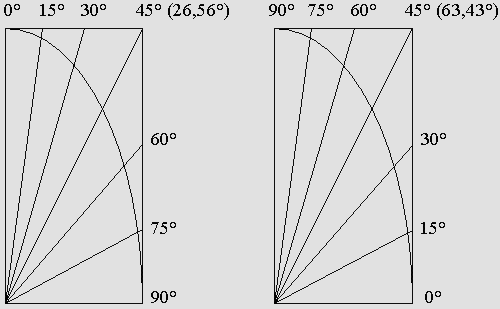The contracted protractor template.     Matter contraction cannot be revealed using triangulation methods. It cannot be revealed using any other method including radar and optical devices either. Even standing wave contraction (see No. 15 below) cannot be revealed because the apparatus also contracts, for example the Michelson interferometer or the screen and receiver Hertz test device. In brief, matter contraction is unverifiable.

 6. THE SPEED OF LIGHT IS APPARENTLY THE SAME IN ALL FRAMES OF REFERENCE. Einstein's most important postulate was (see No. 2 above): "The speed of light is the same in all Galilean frames of reference." Frankly, any reasonable person should realize that this is absurd. However, because all happens as though light really behaves like that, the intelligent scientist (is this a pleonasm?) should firstly consider that he might be fooled. As a matter of fact, there is an explanation for that. The correct postulate should be: "The speed of light is apparently the same whatever the frame of reference where it is measured." Speed is a rather complex notion because it involves both space and time. In addition, a Galilean frame of reference using incompatible space and time units definitely cannot help. Here, one postulates that there is only one Cartesian frame of reference, whose space and time units are absolute. They should be obtained thanks to phenomena which are well known for their invariability. It was the case for the meter, which was firstly established according to a fraction of the earth's meridian, but which is today related to a given wavelength. The time units are still established according the the earth's rotation with respect to the stars. Surely, referring to the earth is somewhat arbitrary, but this convention nevertheless exhibits absolute characteristics. As long as mankind is capable of imposing it on the universe, the universe must adopt it.  According to this convention, the earth (or preferably the center of inertia of the solar system in the case of gravity) is supposed to be stationary as a preferred frame of reference. This is indeed what Lorentz's Relativity admits as a compromise because absolute motion with respect to the aether is not measurable. Thus, we must consider that the speed of light c is 299792458 meters per second, that a second is the time needed for light to travel a 299792458 meters distance and that a meter is the distance which is traveled by light during  1/299792458 of a second. Comparing to other well known invariant phenomena, one finally obtains amazingly accurate - and not transformable - space, time and speed units. Definitely, scientists working on the GPS satellites are not happy with Einstein's Relativity because the idea that each of those satellites must be evaluated according to its own space-time measures is unreasonable. Lorentz's preferred frame of reference appears much better. Any wave emitter which is moving with respect to the earth's surface where the waves are received must use the same space and time units. The waves are obviously suffering from the Doppler effect. It is easily measurable. If the satellite clock and emitter frequency were corrected according to the absolute time, the Doppler effect is more simply contracting the wavelength according to 1 – beta forward and lengthening it according to 1 + beta backward. An astronaut who is orbiting along with a satellite is totally unable to measure the Doppler effect which is modifying the waves emitted by its antenna. However, because of his abnormal situation, he simply cannot postulate that he is stationary in order to explain such a surprising result. He must consider that a Doppler effect does occur, especially because of the Sagnac effect. Quite reasonably, he must admit that he is undergoing the Lorentz transformations in such a way that the Doppler effect becomes undetectable. Let's face it: the speed of light cannot be the same in all frames of reference. A GPS satellite orbiting around the earth cannot be considered to be stationary because it moves faster than the earth whatever the viewpoint. If it is emitting radio waves, their speed cannot be the same forward and backward as measured from any viewpoint. The mere presence of the Doppler effect confirms that the speed of light is not the same in all directions.

7. THERE IS NO WAY TO OBSERVE THAT CLOCKS ARE RUNNING MORE SLOWLY.

 It was seen above that Alice would observe no difference if both the house and she herself had become smaller. Mechanically however, smaller mechanisms should behave faster. For example, a shorter pendulum oscillates faster. But Alice would still be unable to find out that there is an anomaly because her steps, gestures an even metabolism would also occur faster. Alice might be capable of observing a change on the condition that at least one phenomenon behaves differently. The problem is that we are dealing with the Lorentz transformations here. A lot of experiences already proved that they apply to all phenomena without any exception. After more than a century, chances for finding just one which behaves differently are almost nil. On the contrary, the Lorentz transformations become surprisingly visible if the observer is not moving along with a system. But this system may actually be slower than the observer so that the observed transformation is just an illusion. Most probably, our true situation with respect to the aether will remain a mystery forever.

8. THE MICHELSON INTERFEROMETER CANNOT FIND OUT THE ABSOLUTE SPEED.

 This point was discussed in the page on the Michelson Interferometer. More recently, I could release a series of videos on this topic. I had to improve Mr. Marcotte's virtual medium in order to manage the calculation of special devices, for example a moving beam-splitter or a moving parabola. Those videos are a good demonstration that opticians should develop a new science: Motion Optics. It may become an important section of a more general Motion Physics based on the Lorentz transformations.  Please observe the videos below. They show how the light waves should behave in the interferometer. Once again, the results flawlessly lead to the conclusion that Lorentz was right. The contraction proves to be necessary in order to reunify the light beams correctly. The fourth video shows that if there is no contraction, the results are catastrophic. Those images are frankly spectacular! Michelson_Interferometer.5c.mkv Michelson_Interferometer.7c.mkv Michelson_Interferometer_Stationary.mkv Michelson_Interferometer_No_Contraction.mkv Here is the FreeBasic program which produced those sequences: Michelson_Interferometer.bas

9. THE BEAM SPLITTER ANGLE MUST MATCH THE INTERFEROMETER CONTRACTION.

 The videos available above also show that the light beam cannot be reflected correctly unless the beam splitter contracts. If it does not, the reflection angle is not 90° any more. One of the most well known laws in optics stipulates that the reflected angle equals the incident angle. Obviously, according to the new Motion Optics, this law will need a severe upgrade in order to take the contracted protractor template shown above into account. Using the program Ether19.exe   Ether19.bas, I had previously obtained the two diagrams below: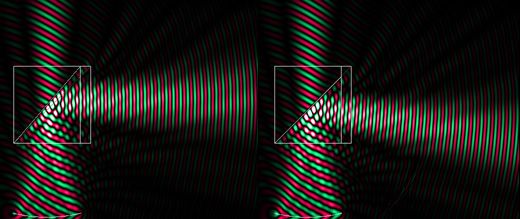Both systems are moving rightward at half of the speed of light. On the left, the convergent beam is deviated correctly by the contracted beam splitter. On the right, the light beam is reflected downward incorrectly if the 45° angle remains unchanged. Here is a video which is more explicit: Michelson_transversal.avi     This video was a world premiere at the time it was created. It evidently proves that the matter contraction hypothesis was not only a convenient and apparently improbable "ad hoc" hypothesis in order to explain Michelson's null result. Now, one must realize that this phenomenon implies a lot of secondary consequences, the most important one being the beam splitter angle modification. One may add the contracted transverse light beam which also exhibits tilted wavefronts, the Doppler effect conciliation and two different time effects (a slower frequency together with a phase wave leading to a time shift) which were carefully analyzed by Lorentz. It appears truly amazing that so many complex phenomena finally prove to behave exactly the way Lorentz predicted. No doubt, he was a genius. Thanks to Mr. Delmotte's amazing virtual medium, which is a powerful laboratory, this experience should be remembered as the most significant one since Michelson's null result. It is even more important because it definitely proves that matter really contracts. It especially proves that the wavefronts propagating transversally become tilted according to the theta angle: theta = arc sin (v / c). For example, if the speed is half of the speed of light as shown above, the theta angle is 30°. It is 45° if beta = 0.707 c. In addition, because of the beam-splitter contraction, the light beam also contracts. I also proved that the Airy disk or the Fresnel-Fraunhofer diffraction pattern contract in the same manner. In brief, this experience proves that the Michelson interferometer must contract. It is unavoidable.

10. BRADLEY'S STELLAR ABERRATION CANNOT REVEAL THE EARTH'S ABSOLUTE MOTION.

 I also created a series of videos on the Bradley stellar aberration. Let's suppose that sun moves with respect to the aether on the same plane as that of the earth's orbit (more or less the ecliptic plane). This configuration supposes that the earth's speed is faster when it moves in the same direction as the sun and that it is slower in the opposite direction. Thus, if a telescope is pointed toward a star whose position is orthogonal every six months, the results should at first glance become asymmetric and finally, reveal the absolute speed of the sun. Bradley's goal was to record a parallax for the nearest stars, but he discovered the stellar aberration instead. But on the contrary, the videos below show that neither the parallax nor the stellar aberration are modified by the absolute motion, at least apparently. Many authors were of an opinion that Bradley's aberration proved that the aether does not exist because the 6-month results are (or more exactly: seem) perfectly symmetrical. So let's be clear: they were wrong! Here, it is important to remind that my Time Scanner is a useful tool which is capable of performing the Lorentz transformations on a given animated scene containing many material bodies. More exactly, it shows how a moving observer should see the scene. I did not correct the contraction because the important point is the symmetry and the simultaneity of the two focal planes. Sorry for this: the correction produces a fuzzy image because pixels are integers. If you prefer, you may stretch the final image according to 1 / g using Photoshop.   The videos show how a moving emitter produces a Doppler effect. In order to reproduce plane waves incoming from very distant stars, I use a parabola which must imperatively be contracted. Otherwise, waves are not plane. Additionally, the plane wavefronts become tilted according to the theta angle. Next, I had to compute two different small parabolas whose speed is not the same as seen above (the 6-month results are performed here by two parabolas moving in opposite direction instead). This is why they are contracted differently. The results are definitely not symmetrical, but the Time Scanner proves that they nevertheless appear symmetrical. The two convergent beams also seem to produce a focus simultaneously. Those are facts. Not hypotheses. They are of the utmost importance for the future. Now, one cannot rely on suppositions and unverifiable arguments about optical phenomena in a moving system. It is all about Motion Optics, a new science which had never been proposed in order to explain Relativity. Motion Optics is a section of the new Motion Physics, which is based on the Lorentz transformations. Starting from now, those experiences will be corroborated by more accurate experiments. You may have to download the DivX codec or try another player (Zoom Player, VLC Media Player) in case of issues. Phase_Wave.mkv        program:  Phase_Wave.bas

11. THE FRESNEL-FRAUNHOFER DIFFRACTION PATTERN CANNOT REVEAL ABSOLUTE MOTION.

 The Fresnel diffraction pattern is well known. As far as I know, nobody ever showed how it should behave if the source was moving, though. Thanks to his awesome virtual medium, Mr. Delmotte released the image below. The linear source is 12 wavelengths wide: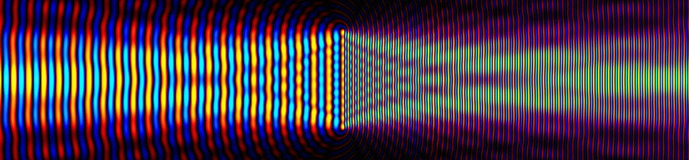Because of the Doppler effect, it was predictable that the wavelength should contract forward and expand backward. However, the fact that the general diffraction pattern is identical on both sides is a surprise. What's more, the pattern on both sides contracts according to Lorentz predictions. The wavelength difference is not noticeable either because measuring the wavelength while moving is not possible without firstly producing standing waves by means of a flat mirror or reflector. The Hertz test or an interferometer actually measures the node and antinode position, which is the same on both sides. And finally, it will be seen below (No. 15), those standing waves contract. Surely, if the observer is moving along with this emitter, he is unable to notice the pattern contraction and the wavelength contraction because he himself is contracted.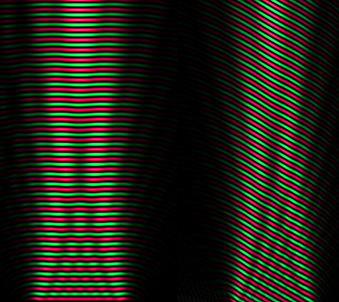On the left hand side, the unmoving source produces the regular 2-D Fresnel diffraction pattern. The source on the right emits a wave beam transversally at half of the speed of light.     One may compare the modified diffraction pattern to the normal one. It is seen that the wavefronts are tilted to the theta angle: theta = arc sin(beta) = 30° because the waves actually propagate according to this angle. This angle cannot be checked out because of the time shift. The number of wavelengths (30 here from top to bottom) does not change but the pattern contracts on the axis x according to Lorentz's predictions. Finally, whatever the orientation, the pattern seems unchanged from the observer's viewpoint.

12. RELATIVE MOTION ONLY IS MEASURABLE.

 Lorentz predicted that the frequency should slow down according to the contraction factor g. As a result, the Doppler effect forward and backward becomes perfectly symmetrical. Because of this, absolute motion is unverifiable, for example that of a distant galaxy. But the observer can still measure its relative speed. As a matter of fact, astronomers presume that distant galaxies are moving away from our own galaxy, but one should bear in mind that our galaxy may be moving away from them as well. Let's suppose that a plane is moving away at half of the speed of the sound (beta = 0.5; g = 0.866). Then the sound waves emitted by the plane undergo a normal (non relativistic) Doppler effect: The forward wavelength is:  1 – beta  =  0.5 times shorter. The backward wavelength is:  1 + beta  =  1.5 times longer.    There is no symmetry: 0.5 * 1.5 = 0.75. But in the case of light however, the emitter frequency slows down according to g. The forward wavelength is:  (1 – beta) / g  =  0.577 times shorter. The backward wavelength is :  (1 + beta) / g  =  1.732 times longer. The result is a surprisingly symmetric Doppler effect which prevents the astronomer to measure the absolute speed of a galaxy. 0.577 * 1.732 = 1 Such a result is a remarkable characteristic of the Lorentz transformations. Poincare said about matter contraction: "This strange property would seem to be a true thumb snap (a helpful hand) from nature in order to avoid the Earth's motion being revealed by optical phenomena. This cannot satisfy me and I must express here my feeling: it looks as if optical phenomena are very likely dependent on relative motion between material bodies." But actually, the Lorentz symmetric Doppler effect is far more misleading. It was seen that matter contraction, the slower time and the local hours could fool the moving observer, but this additional symmetry is somewhat diabolic. The result is that nobody can say for sure what is going on. Motion appears purely relative and hence, anybody is entitled to presume that he is stationary. The speed of any moving body is measured with respect to him. Such a presumption may lead to unreasonable ideas, though. All this is terribly clever, magical, magnificent, if not supernatural. But the dark side is that mankind may never know the truth about his origin and his destination in space and time.

13. ANY SPEED BEYOND THE SPEED OF LIGHT IS IMPOSSIBLE.

 Lorentz and Poincare discovered that the speed of light is an unattainable limit for matter because its mass grows up according to the gamma factor. Mass being the measure of inertia, it would becomes infinite at the speed of light. Lorentz's reasoning was correct, but it was not quite convincing. Today however, because matter is made out of waves, the possibility that anything whatsoever could move faster than the speed of light becomes nil. The real cause of the increase in mass is the Doppler effect and I could prove this by means of active and reactive mass, which also explain action and reaction effects. The nature of matter waves is the same as that of all forces including light. Their speed being the speed of light, absolutely nothing can travel faster. I am rather surprised that so many authors are invoking Lorentzian Relativity in order to explain marginal effects such as EPR. In my opinion, a delayed effect may very well explain apparently instantaneous action as long as the cause influences two particles simultaneously and exactly in the same manner. The point is that a field of force always influences two particles simultaneously because it is standing in the center. It is invisible and hence it was ignored when scientists tried to explain those apparently strange phenomena. What's more, they are frequently explained differently and this proves that the conclusions are not so convincing. Lorentz never admitted faster than light speeds. Consequently, Lorentzian Relativity denies such phenomena.

14. THE MAXIMUM RELATIVE SPEED IS TWICE THE SPEED OF LIGHT.

 The main problem with Einstein's Relativity is that it cannot compare more than two frames of reference. Worse, the results are contradictory, as the twin paradox clearly shows. Einstein's supporters try to complicate the problem by introducing an acceleration or a deceleration. But if their speed is not the same and if their inertia is never modified, the real question is: "which one is getting older?" On the contrary, by introducing a preferred frame of reference, Lorentz's Relativity always remain perfectly logical and coherent even in the presence of many material bodies moving at any speed and in any direction. Some of these may appear severely transformed, hence less privileged. Fortunately, thanks to my Time Scanner, I can transform the whole scene the way it would appear as seen from any of these: Time_Scanner.mkv This video is the ultimate proof that transforming space and time was not a good idea. Transforming matter works best. In addition, the video shows a red scale which does not transform at all. Clearly, the less something is transformed, the more it is a good choice in order to establish a preferred frame of reference. This might be the case for the center of inertia of a complex mechanism, that of the solar system for example. The distortion being minimum there, it should yield more accurate results concerning Mercury's orbit. One should also avoid Poincare's famous "chaos" theory because, in my opinion, any surrealist result is simply the consequence of a wrong calculus. The video shows two systems moving in opposite direction at half the speed of light. Because each of these may be accelerated to nearly the speed of light, it becomes clear that their relative speed could be nearly twice the speed of light. It would be the case for two electrons moving in opposite directions in a giant collider. Thanks to Lorentz's Relativity, which accepts an unlimited number of frames of reference, the collider can be added as a preferred unmoving frame of reference. It is the best place for the scientist to examine the situation. On the contrary, another observer moving along with one of those electrons would observe a severely distorted scene where the fast moving collider itself would seem strongly contracted and where the other electron would still seem to move only at nearly the speed of light. Not twice. Obviously, even such incompatible results are preferable because the worst of these may be rejected. From an absolute point of view, however, one should bear in mind that even the apparently most distorted viewpoint may possibly be the correct one. Certitude may be compared to the speed of light: it is unreachable, but one may approach it closely.

15. STANDING WAVES CONTRACT TOGETHER WITH A SLOWER FREQUENCY.

This was demonstrated at the page on plane standing waves, albeit spherical standing waves and especially my moving electron behave this way too. I will just suggest to examine the images below because they beautifully show that.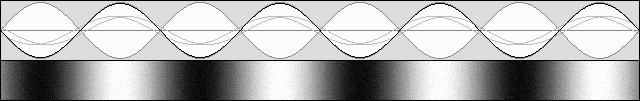Truly "standing" waves.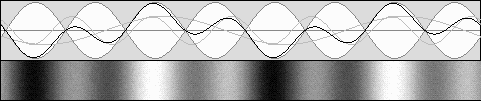The system speed is half of the speed of light (v = 0.5 c; g = 0.866)

The frequency being unchanged, a node and antinode contraction occurs according to g squared:  0.866 2 = 0.75.

Here, the observer is stationary with respect to the aether.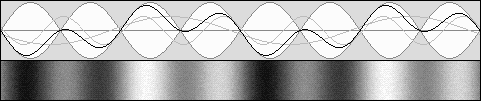This is the same system but now the observer is moving along with it so that it seems stationary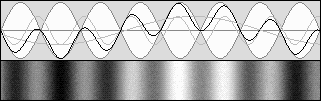Here, the speed was increased to sin 45° = 0.707 c  (g = cos 45° = 0.707 equally).

The frequency being unchanged, the axial contraction is: g 2 = 0.5.

However, according to Lorentz, the frequency should slow down according to g.

That is why standing waves finally contract axially according to g, not g squared.

On a transverse axis, the wavelength normally contracts according to Lorentz's contraction factor g as a consequence of the normal Doppler effect (no frequency shift). However, one may obtain transverse standing waves using two parallel flat screens. In this case, the resonance frequency slows down according to g on the condition that the screen distance remains unchanged. Finally, the slower frequency corrects the standing wave contraction and the number of nodes and antinodes between the screens remains constant.

Because matter is made of standing waves, it contracts in the direction of motion according to x' = g x. But it does not contract on orthogonal axes: y'=y; z'=z. This is possible because the electron frequency slows down according to t' = g t. It becomes clear that all this is highly consistent with the Lorentz transformations:

 x' =  g x + b t t'  =  g t – b x y' = y    z' = z

But unfortunately, Lorentz was not aware of this amazing standing wave behavior.

16. LORENTZIAN RELATIVITY REMAINS CONSISTENT IN THE PRESENCE OF MANY MATERIAL BODIES.

 Please examine again the video below, which was proposed in the beginning of this page: Time_Scanner.mkv As I previously said, such a magnificent harmony should leave nobody unfeeling. Einstein's method is hard to figure out because each material body belongs to a separate space-time system, and additionally the results are contradictory. Lorentz's viewpoint is much better. Not only did I succeed in showing many systems in the same image, the results are also transposed as a whole into another frame of reference without any discrepancy. The Time Scanner can show how the whole scene should appear as seen from any observer whatever his speed. Clearly, creating a separate frame of reference for each object is not an option. It is simply ridiculous. The great advantage of Lorentzian Relativity is its logic. It is not a compilation of obscure, dogmatic and inviolable principles, it is highly explainable. It is founded on Euclidean geometry and on Descartes' space system. At last, one can understand what is going on, and there are no paradoxes any more.

17. THERE IS NO GENERAL RELATIVITY BECAUSE GRAVITY IS NOT LINKED TO RELATIVITY.

 Gravity is not the fundamental force of the universe. This study indicates that it is caused by the same sort of waves which are responsible for all forces. Gravity is definitely an ordinary force. It is not fundamentally different from the Lorentz force or the Coulomb force, for example. An observer being accelerated in an elevator cage by a "good little devil" (this image was borrowed from Maxwell) indeed feels as if he was experiencing gravity. However, any other equivalent force applied to the cage would produce exactly the same effect. Also, any material body highly compressed because of gravity, acceleration or deceleration (or by any other force such as centrifuge) would certainly behave differently for mechanical reasons. A clock would obviously show modified hours, but such a result is certainly not a "time transformation". And incidentally, gravity does not bend space. This idea is the most ridiculous ever. In brief, it is about time to come back down to earth. Whatever the situation where Relativity is to be considered, all forces including gravity behave in the same manner.

18.  THE LORENTZ TRANSFORMATIONS FIRSTLY LEAD TO RELATIVITY BUT EXTEND WELL BEYOND IT .

 The Lorentz transformations apply to matter and to aether waves as a Doppler effect in such a way that our absolute motion through the aether is not measurable. This is the most stunning consequence, but it is not the most important. It should be emphasized that we are mainly dealing with matter mechanics in the presence of motion. The new mechanics as proposed by Henri Poincare is actually the Motion Physics. The Lorentz transformations will allow us to rewrite the whole physics and to elaborate new laws which will at last become perfectly true forever. At first glance, it appears advisable to upgrade Newton's laws because they are highly understandable and familiar. After a century of Relativity, scientists still rely on Newton's laws even though they are aware that their accuracy is problematic. They feel that Newton was not totally wrong. The challenge for the next century will be to upgrade his work. I already started the project but hundreds of physicists and quite a long time will be needed until it is complete.| 01 | 02 | 03 | 04 | 05 | 06 | 07 | 08 | 09 | 10 | You are here | 12 | 13 | 14 | 15 | 16 |

| 17 | 18 | 19 | 20 | 21 | 22 | 23 | 24 | 25 | 26 | 27 | 28 | 29 | 30 | 31 | 32 | 33 | 34 |

 Gabriel LaFreniere, Bois-des-Filion in Québec. On the Internet since September 2002. Last update March 11, 2011.

 Matter is made of Waves The electron Ivanov's Waves Spherical Standing Waves The Doppler Effect The Aether The Michelson Interferometer The Lorentz Transformations The Time Scanner Lorentzian Relativity Page 1 Lorentzian Relativity Page 2 The Wave Mechanics Electrostatic Fields Nuclear Forces Active and Reactive Mass Kinetic Energy Fields of Force The Fields of Force Dynamics Magnetic Fields Gravity Protons Atoms Chemistry The Wave Theory The Wave Theory Postulates The Theory of Evolution Errors to Correct Proofs and Experiences The Huygens Principle